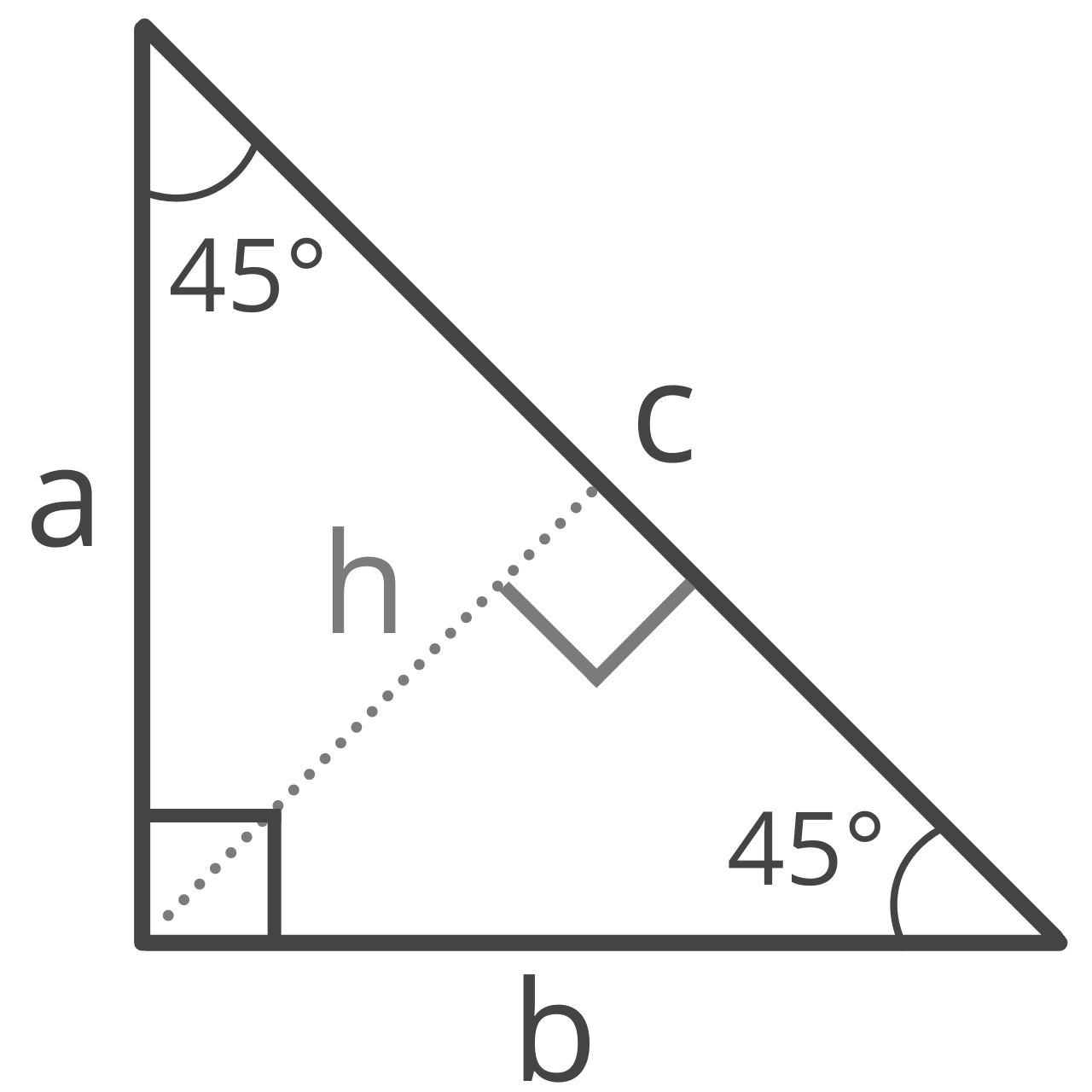# 45 45 90 Triangle Calculator

Enter any known value for a 45 45 90 triangle to calculate the edge lengths, altitude, area, perimeter, inradius, and circumradius.## Triangle Properties:

 leg a: 3 leg b: 3 hypotenuse c: 4.243 height h: 2.121 area: 4.5 perimeter: 10.243 inradius: 0.8787 circumradius: 2.121
Learn how we calculated this below

## What is a 45 45 90 Triangle?

A 45 45 90 triangle is a special right triangle that has two 45° interior angles and one 90° right angle. In addition to being a right triangle, a 45 45 90 triangle is also an isosceles triangle.

You can also think of a 45 45 90 triangle as half of a square divided from one corner to the opposite corner.

Like all triangles, the side lengths of a 45 45 90 triangle correspond with the size of the angle opposite the side. The side length opposite the 90° angle will be the longest side.

The two remaining side lengths, in addition to being shorter than the side length opposite the 90° angle, are both opposite a 45° angle and will therefore be equal to each other.## Formulas to Solve a 45 45 90 Triangle

In a 45 45 90 triangle, the ratio of the side lengths is 1 : 1 : √2. Keep in mind this ratio is structured as a : b : c, where a and b are the two shorter side lengths opposite the 45° angle (often called the legs), and c is the longest side length (called the hypotenuse).

Using this ratio, you can solve for any side length if you know just one of the other side lengths. The phrase “solving a triangle” typically refers to using given information to find unknown side lengths and angles.

Note, this ratio can help you evaluate the sine, cosine, and tangent of either of the 45° angles of a 45 45 90 triangle.

The sine of an angle is equal to the side length opposite the angle divided by the hypotenuse, the cosine of an angle is equal to the side length adjacent to the angle divided by the hypotenuse, and the tangent of an angle is equal to the side length opposite the angle divided by the side length adjacent to the angle.

Since the ratios never change, regardless of the lengths of the three sides, the values of sine, cosine, and tangent evaluated at 45° will always be the same.

The tangent of the 45° angle is equal to its adjacent side divided by the opposite side, which is equal to 1.

sin(45°) = 1 / √2

cos(45°) = 1 / √2

tan(45°) = 1

### Hypotenuse Formulas

Because a 45 45 90 triangle is a right triangle, you can use the Pythagorean theorem to solve for unknown side lengths. In addition to the Pythagorean theorem, there are also a few simplified formulas that can be used on a 45 45 90 triangle as well, which allow you to solve for unknown side lengths given only one side.

The Pythagorean Theorem is typically expressed as:

a² + b² = c²

Where a and b are the two legs of the right triangle, and c is the hypotenuse. However, the equation can be manipulated algebraically to isolate the hypotenuse, c.

Thus, the formula to solve the hypotenuse is:

c = a² + b²

The hypotenuse c is equal to the square root of leg a squared plus leg b squared. Note that in a 45 45 90 triangle legs a and b are the same length.

However, since the ratio of the short legs to the hypotenuse in a 45 45 90 triangle is 1 : √2, the following is a simple formula to calculate the length of the hypotenuse:

c = a√2

The hypotenuse c is equal to leg a times the square root of 2.

You can also find this using our hypotenuse calculator.

### Leg Length Formulas

Given the hypotenuse and using the 1 : √2 ratio, the length of the legs can be solved using the following formula:

a = b = c√2 / 2

The legs a and b are equal to the hypotenuse c times the square root of 2, divided by 2.

Alternatively, you can find the length of the legs if you know the hypotenuse, given that the interior angles are known to be 45°. This is the formula for this method:

a = b = c × sin(45°)

The legs a and b are equal to the hypotenuse c times the sine of the 45° angle.

### Area Formula

The area of a 45 45 90 triangle can be solved with the formula:

A = / 2 = / 2

Thus, the area A is equal to the length of leg a squared (or leg b squared), divided by 2

### Perimeter Formula

Use this formula to calculate the perimeter:

P = 2a + c = 2b + c

Thus, the perimeter P is equal to 2 times leg a (or leg b) plus hypotenuse c.

For more special right triangle formulas, try our 30 60 90 calculator.